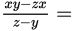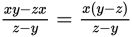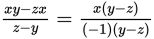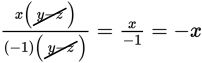# SAT Math Multiple Choice Question 894: Answer and Explanation

### Test Information

Question: 894

9. If yz, then• A. -x
• B. -1
• C. 1
• D. x

Explanation:

A

Difficulty: Easy

Category: Passport to Advanced Math / Exponents

Strategic Advice: Whenever you need to simplify a fraction that involves binomials, your first thought should be: factor!

Getting to the Answer: Because x is in both terms of the numerator, factor out x to get:Next, rewrite (z - y) as -1(y - z) to get:The numerator and denominator now share a common factor, y - z, which can be cancelled:The result matches (A).